# Simulation Model

In this tutorial, we'll demonstrate the steps to construct one 12-month sales simulation using an ARIMA(5,1,1) model. Next, using the simulated 12-month (step) values, we'll compute their median as an example of a target (derived) value. Finally, using the "Run" functionality, NumXL will re-evaluate the spreadsheet (creating a new simulation path each time) and collect the target values in a separate column in our spreadsheet. Once done, we are left with several potential values for the median of the following 12 sales months and may start analyzing them as we see fit.

## Process - Part 1

1. Select the upper cell of a given model table.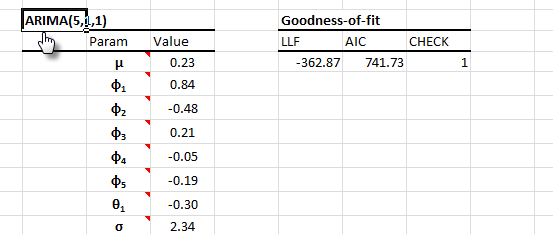2. Locate the "Simulation" icon in the NumXL toolbar (or menu in Excel 2003) and click it.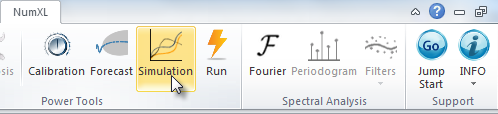3. The Simulation Wizard (dialog box) appears. Note that the model field in the dialog references the active (selected) cell in your worksheet.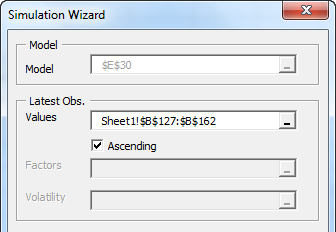4. Now, select the latest (or the whole set) observations in your input data. In this example, we chose 35 observations.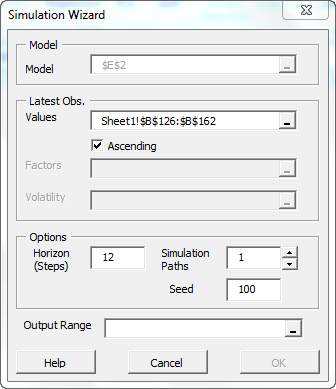5. In the "Horizon" field, we chose 12 steps for one 12-month simulation path.
6. Leave the seed to 100.
7. In the "Output Range" field, select an empty cell in your workbook to store the simulation path.
8. Click "OK".

## Output: Part 1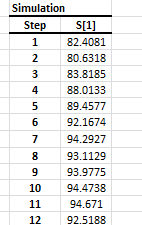We have now managed to construct one 12-month simulation path for an ARIMA(5,1,1) model and the latest observations in our data set.

Next, let's assume we wish to calculate a value that uses one or more observations in the simulation path. For this tutorial, we will compute the median of the monthly sales for the following year. Using one simulation path, we compute only one value. Hitting F9 will cause NumXL to generate new values for the simulation path, forcing re-evaluation, and therefore generating a new target value.

What if we want to use 100 simulation paths and collect the target value of each path? We can instruct the Simulation Wizard to generate 100 paths, then compute the target value for each path. Sounds easy enough, but what about 10,000 simulation paths?

We can make this simpler by generating one simulation path, calculating the target value, then using the Run Wizard to make NumXL re-generate the same simulation path as many times as we wish. Then we collect the target value for each run and store the values in your worksheet.

## Process: Part 2

Here are the steps:

1. Select an empty cell in your worksheet where you wish the output to be displayed.
2. Locate the Run icon in the NumXL toolbar (or menu in Excel 2003) and click it.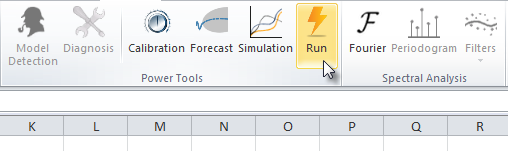3. The Simulation Run Wizard pops up. By default, the output references the active (selected) cell in your workbook.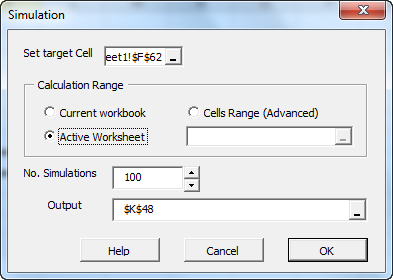4. Next, select the cell for the target value.
5. Now, we need to decide the extent of the re-calculation needed to evaluate the target value cell. For our tutorial, we'll choose "Active Worksheet".
Note: If you are unsure or if the calculation spans multiple worksheets, select "current workbook".
6. Finally, set the number of simulations to a value (less than 30,000) for reasonable performance. We'll choose 100 (default) for this tutorial.
7. Click "OK".

## Output: Part 2

The Run Wizard generates the values for each simulation run.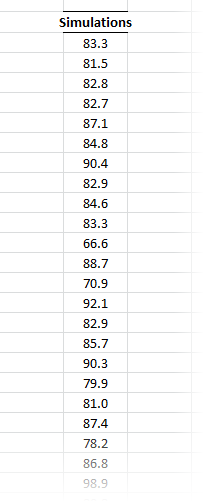The computed target values of the different simulation runs are statistically independent, so you may wish to run summary statistics (e.g. mean, standard deviation, etc.) or plot their empirical distribution.

For this tutorial, we chose a histogram plot: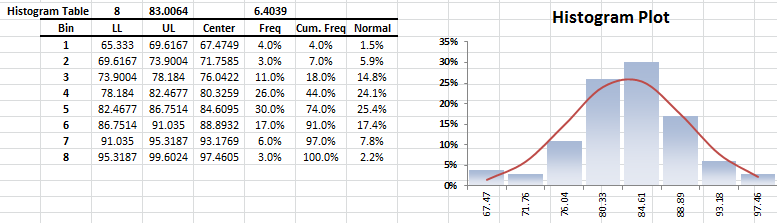Run the normality test.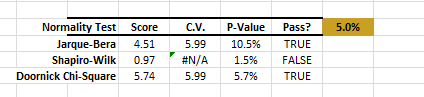Q: Do I need to use the NumXL simulation functions to use the Run Wizard?
A: No! If you hit F9 and your target cell value is refreshed, then you can use the Run Wizard for any type of simulation.